# Class 10 Mathematics: CBSE Sample Question Paper (2017-18) Notes | Study CBSE Sample Papers For Class 10 - Class 10

## Class 10: Class 10 Mathematics: CBSE Sample Question Paper (2017-18) Notes | Study CBSE Sample Papers For Class 10 - Class 10

The document Class 10 Mathematics: CBSE Sample Question Paper (2017-18) Notes | Study CBSE Sample Papers For Class 10 - Class 10 is a part of the Class 10 Course CBSE Sample Papers For Class 10.
All you need of Class 10 at this link: Class 10

General Instructions:

• All questions are compulsory.
• The question paper consists of 30 questions divided into four sections A, B, C and D.
• Section A contains 6 questions of 1 mark each. Section B contains 6 questions of 2 marks each. Section C contains 10 questions of 3 marks each. Section D contains 8 questions of 4 marks each.
• There is no overall choice. However, an internal choice has been provided in four questions of 3 marks each and three questions of 4 marks each. You have to attempt only one of the alternatives in all such questions.
• Use of calculators is not permitted.

Section A

Question numbers 1 to 6 carry 1 mark each.

Q.1) Write whether the rational number 7/75 will have a terminating decimal expansion or a nor-terminating repeating decimal expansion.

Q.2) Find the value(s) of k, if the quadratic equation 3x2 k √3x + 4 =0 has equal roots.

Q.3) Find the eleventh term from the last term of the AP: 27, 23, 19, ..., –65.

Q.4) Find the coordinates of the point on y-axis which is nearest to the point (–2, 5).

Q.5) In given figure, ST || RQ, PS = 3 cm and SR = 4 cm. Find the ratio of the area of Δ PST to the area of Δ PRQ.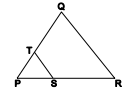Q.6) If cos A  =2/5, find the value of 4 + 4 tan2 A

Section B

Question numbers 7 to 12 carry 2 marks each.

Q.7) If two positive integers p and q are written as p = a 2 b3 and q= a3b; a, b are prime numbers, then verify:

LCM (p, q) × HCF (p, q) = pq

Q.8) The sum of first n terms of an AP is given by S= 2n2+ 3n . Find the sixteenth term of the AP.

Q.9) Find the value(s) of k for which the pair of linear equations kx + y = k2 and x + ky = 1 have infinitely many solutions.

Q.10) If (1, P/5) is the mid-point of the line segment joining the points (2, 0) and (0,2/9), then show that the line 5x + 3y + 2 = 0 passes through the point (–1, 3p).

Q.11) A box contains cards numbered 11 to 123. A card is drawn at random from the box. Find the probability that the number on the drawn card is
(i) a square number
(ii) a multiple of 7

Q.12) A box contains 12 balls of which some are red in colour. If 6 more red balls are put in the box and a ball is drawn at random, the probability of drawing a red ball doubles            than what it was before. Find the number of red balls in the bag.

Section C

Question numbers 13 to 22 carry 3 marks each.

Q.13)  Show that exactly one of the numbers n, n + 2 or n + 4 is divisible by 3.

Q.14) Find all the zeroes of the polynomial 3x4 + 6x3 - 2x2 - 10x - 5 if two of its zeroes are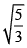and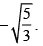Q.15) Seven times a two digit number is equal to four times the number obtained by reversing the order of its digits. If the difference of the digits is 3, determine the number.

Q.16) In what ratio does the x-axis divide the line segment joining the points (–4, –6) and (–1, 7)? Find the co-ordinates of the point of division.

OR

The points A(4, –2), B(7, 2), C(0, 9) and D(–3, 5) form a parallelogram. Find the length of the altitude of the parallelogram on the base AB.

Q.17) In given figure ∠1 = ∠2 and ΔNSQ ≌ ΔMTR , then prove that ΔPTS ~DPRQ .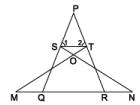In an equilateral triangle ABC, D is a point on the side BC such that BD = 1/3 BC. Prove that  9AD2 = 7AB2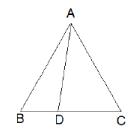Q.18) In given figure XY and X'Y' are two parallel tangents to a circle with centre O and another tangent AB with point of contact C intersecting XY at A and X'Y' at B. Prove                that ∠ AOB = 90°.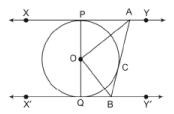Q.19) Evaluate: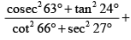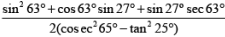OR

If sin θ+ cos θ =√2 then evaluate : tan θ + cot θ

Q.20) In given figure ABPC is a quadrant of a circle of radius 14 cm and a semicircle is drawn with BC as diameter. Find the area of the shaded region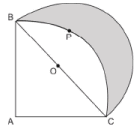Q.21) Water in a canal, 6 m wide and 1.5 m deep, is flowing with a speed of 10 km/h. How much area will it irrigate in 30 minutes, if 8 cm of standing water is needed?

OR

A cone of maximum size is carved out from a cube of edge 14 cm. Find the surface area of the remaining solid after the cone is carved out.

Q.22) Find the mode of the following distribution of marks obtained by the students in an examination:

 Marks obtained 0-20 20-40 40-60 60-80 80-100 Number of students 15 18 21 29 17

Given the mean of the above distribution is 53, using empirical relationship estimate the value of its median.

Section D

Question numbers 23 to 30 carry 4 marks each.

Q.23) A train travelling at a uniform speed for 360 km would have taken 48 minutes less to travel the same distance if its speed were 5 km/hour more. Find the original speed              of the train.

OR

Check whether the equation 5x2 – 6x – 2 = 0 has real roots and if it has, find them by the method of completing the square. Also verify that roots obtained satisfy the given equation.

Q.24) An AP consists of 37 terms. The sum of the three middle most terms is 225 and the sum of the last three terms is 429. Find the AP.

Q.25) Show that in a right triangle, the square of the hypotenuse is equal to the sum of the squares of the other two sides.

OR

Prove that the ratio of the areas of two similar triangles is equal to the ratio of the squares of their corresponding sides.

Q.26) Draw a triangle ABC with side BC = 7 cm, ∠B = 45°, ∠A = 105° . Then, construct a triangle whose sides are 4/3 times the corresponding sides of ΔABC.

Q.27) Prove that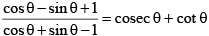Q.28) The angles of depression of the top and bottom of a building 50 metres high as observed from the top of a tower are 30° and 60°, respectively. Find the height of the tower and also the horizontal distance between the building and the tower.

Q.29) Two dairy owners A and B sell flavoured milk filled to capacity in mugs of negligible thickness, which are cylindrical in shape with a raised hemispherical bottom. The                  mugs are 14 cm high and have diameter of 7 cm as shown in given figure. Both A and B sell flavoured milk at the rate of Rs 80 per litre. The dairy owner A uses the                    formula πr2h to find the volume of milk in the mug and charges Rs 43.12 for it. The dairy owner B is of the view that the price of actual quantity of milk should be                        charged. What according to him should be the price of one mug of milk? Which value is exhibited by the dairy owner B? (use π = 22/7)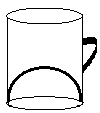Q.30) The following distribution shows the daily pocket allowance of children of a locality. The mean pocket allowance is Rs18. Find the missing frequency k.

 Daily pocket allowance (in Rs) 11–13 13–15 15–17 17–19 19–21 21–23 23–25 Number of children 3 6 9 13 k 5 4

OR

The following frequency distribution shows the distance (in metres) thrown by 68 students in a Javelin throw competition.

 Distance (in m) 0–10 10–20 20–30 30–40 40–50 50–60 60–70 Number of students 4 5 13 20 14 8 4

Draw a less than type Ogive for the given data and find the median distance thrown using this curve.

The document Class 10 Mathematics: CBSE Sample Question Paper (2017-18) Notes | Study CBSE Sample Papers For Class 10 - Class 10 is a part of the Class 10 Course CBSE Sample Papers For Class 10.
All you need of Class 10 at this link: Class 10Use Code STAYHOME200 and get INR 200 additional OFF

## CBSE Sample Papers For Class 10

174 docs|7 tests

Track your progress, build streaks, highlight & save important lessons and more!

,

,

,

,

,

,

,

,

,

,

,

,

,

,

,

,

,

,

,

,

,

;